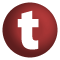# PartialOrdering

#### trait PartialOrdering[T] extends Equiv[T]

A trait for representing partial orderings. It is important to distinguish between a type that has a partial order and a representation of partial ordering on some type. This trait is for representing the latter.

A partial ordering is a binary relation on a type `T` that is also an equivalence relation on values of type `T`. This relation is exposed as the `lteq` method of the `PartialOrdering` trait. This relation must be:

• reflexive: `lteq(x, x) == true`, for any `x` of type `T`.
• anti-symmetric: `lteq(x, y) == true` and `lteq(y, x) == true` then `equiv(x, y)`, for any `x` and `y` of type `T`.
• transitive: if `lteq(x, y) == true` and `lteq(y, z) == true` then `lteq(x, z) == true`, for any `x`, `y`, and `z` of type `T`.

known subclasses: Ordering

1. Equiv
2. AnyRef
3. Any

### Value Members

1. #### def equals(arg0: Any): Boolean

This method is used to compare the receiver object (`this`) with the argument object (`arg0`) for equivalence

This method is used to compare the receiver object (`this`) with the argument object (`arg0`) for equivalence.

The default implementations of this method is an equivalence relation:

• It is reflexive: for any instance `x` of type `Any`, `x.equals(x)` should return `true`.
• It is symmetric: for any instances `x` and `y` of type `Any`, `x.equals(y)` should return `true` if and only if `y.equals(x)` returns `true`.
• It is transitive: for any instances `x`, `y`, and `z` of type `AnyRef` if `x.equals(y)` returns `true` and `y.equals(z)` returns `true`, then `x.equals(z)` should return `true`.

If you override this method, you should verify that your implementation remains an equivalence relation. Additionally, when overriding this method it is often necessary to override `hashCode` to ensure that objects that are "equal" (`o1.equals(o2)` returns `true`) hash to the same `Int` (`o1.hashCode.equals(o2.hashCode)`).

arg0

the object to compare against this object for equality.

returns

`true` if the receiver object is equivalent to the argument; `false` otherwise.

definition classes: AnyRef ⇐ Any
2. #### def equiv(x: T, y: T): Boolean

Returns `true` iff `x` is equivalent to `y` in the ordering

Returns `true` iff `x` is equivalent to `y` in the ordering.

3. #### def gt(x: T, y: T): Boolean

Returns `true` iff `y` comes before `x` in the ordering and is not the same as `x`

Returns `true` iff `y` comes before `x` in the ordering and is not the same as `x`.

4. #### def gteq(x: T, y: T): Boolean

Returns `true` iff `y` comes before `x` in the ordering

Returns `true` iff `y` comes before `x` in the ordering.

5. #### def hashCode(): Int

Returns a hash code value for the object

Returns a hash code value for the object.

The default hashing algorithm is platform dependent.

Note that it is allowed for two objects to have identical hash codes (`o1.hashCode.equals(o2.hashCode)`) yet not be equal (`o1.equals(o2)` returns `false`). A degenerate implementation could always return `0`. However, it is required that if two objects are equal (`o1.equals(o2)` returns `true`) that they have identical hash codes (`o1.hashCode.equals(o2.hashCode)`). Therefore, when overriding this method, be sure to verify that the behavior is consistent with the `equals` method.

definition classes: AnyRef ⇐ Any
6. #### def lt(x: T, y: T): Boolean

Returns `true` iff `x` comes before `y` in the ordering and is not the same as `y`

Returns `true` iff `x` comes before `y` in the ordering and is not the same as `y`.

7. #### def lteq(x: T, y: T): Boolean

Returns `true` iff `x` comes before `y` in the ordering

Returns `true` iff `x` comes before `y` in the ordering.

attributes: abstract

9. #### def toString(): String

Returns a string representation of the object

Returns a string representation of the object.

The default representation is platform dependent.

definition classes: AnyRef ⇐ Any
10. #### def tryCompare(x: T, y: T): Option[Int]

Result of comparing `x` with operand `y`

Result of comparing `x` with operand `y`. Returns `None` if operands are not comparable. If operands are comparable, returns `Some(r)` where `r < 0` iff `x < y` `r == 0` iff `x == y` `r > 0` iff `x > y`

attributes: abstract